Try NerdPal! Our new app on iOS and Android

# Determine whether the expression $ir^2x\sqrt{y}$ is rational

## Related Videos

Go!
Go!
1
2
3
4
5
6
7
8
9
0
a
b
c
d
f
g
m
n
u
v
w
x
y
z
.
(◻)
+
-
×
◻/◻
/
÷
2

e
π
ln
log
log
lim
d/dx
Dx
|◻|
θ
=
>
<
>=
<=
sin
cos
tan
cot
sec
csc

asin
acos
atan
acot
asec
acsc

sinh
cosh
tanh
coth
sech
csch

asinh
acosh
atanh
acoth
asech
acsch

### Videos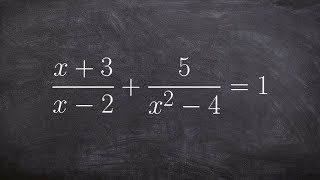### Algebra 2 - Learning to solve rational equations in math class ((x+3)/(x‐2)) + (5/(x^2‐4)) = 1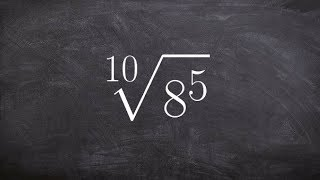### Algebra 2 - Simplifying a radical expression to rational exponents, tenthroot ( 8^5)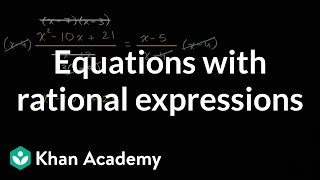### Equations with rational expressions | Mathematics III | High School Math | Khan Academy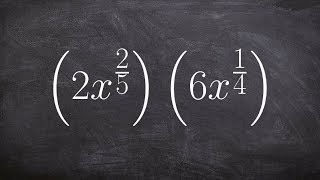### Algebra 2 - Multiplying two exponents with rational powers, then simplifying, 2x^(2/5) . 6x^(1/4)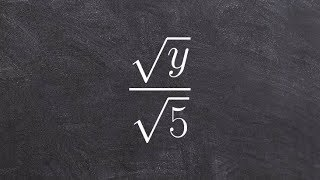### Algebra 2 - Learn how to rationalize the denominator to simplify an expression, sqrt(y)/sqrt(5)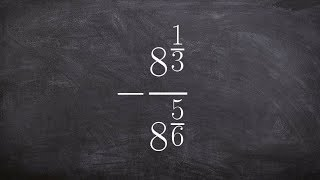### Algebra 2 - Learn how to divide two exponents with rational powers -(8^(1/3))/(8^(5/6))

$rational\left(i r^2\cdot x\sqrt{y}\right)$